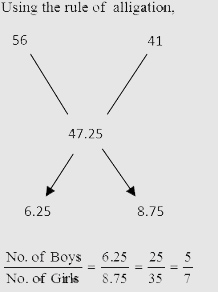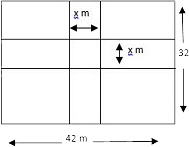# Sample Aptitude Questions of Genpact

DIRECTIONS for the question 1 to 2: Solve the following question and mark the best possible option.
1. Relationship between x and y cannot be established
2. If x > y
3. If x < y
4. If x ≥ y
5. If x ≤ y
1. 10x2 + 11x + 1 = 0
II. 12y2 - y - 1 = 0
1. 1
2. 2
3. 3
4. 4
5. 5
I. 10x2 + 11x + 1 = 0
=> x = -1 , -1/10
II. 12y2 - y - 1 = 0
=> y = 1/3 , -1/4
For x = - 1 , -1 < 1/3 and -1 < -1/4
For x = - 1/10 , -1/10 < 1/3 but -1 < -1/10 > -1/4
So no unique relationship between x and y. Hence 1st option.
1. x2 - 14x - 51 = 0
II. y2 - 35y + 306 = 0
1. 1
2. 2
3. 3
4. 4
5. 5
I. x2 - 14x - 51 = 0
=> x = 17 , -3
II. y2 - 35y + 306 = 0
=> y = 18 , 17
For : x = 17
=> 17 < 18 , 17 = 17
For x = -3 => -3 < 18 , -3 < 17
So we get x ≤ y.
DIRECTIONS for the questions 3 to 4: In the following series, one of the terms given is wrong. Find that term and mark that as your answer
2. 40 20 24 30 60 150
1. 24
2. 60
3. 20
4. 150
5. 30
The logic is ×0.5 , ×1 , ×1.5, ×2 ,×2.5 …..
So following the logic we get 24 is the wrong number and instead it should be 20. Hence 1st option.
3. 29 33 41 50 77 98 157
1. 33
2. 77
3. 50
4. 98
5. 41
The logic is, +4, +8, +9, 27, +16, +64,….
i.e. +22,+23,+32,+33,+42,+43,…..
So following the logic we get that 98 is wrong . So Ans is (D)
4. What is the percent profit earned by selling the article after giving 10% discount? I. Had there been no discount offered, the profit earned would have been 30%. II. Selling price of the article after giving discount is Rs. 3510/-
1. The data in statement II alone are sufficient to answer the question, while the data in statement I alone are not sufficient to answer the question.
2. The data either in statement I alone or in statement II alone are sufficient to answer the question.
3. The data in both the statement I & II together are not sufficient to answer the question.
4. The data in both the statements I & II together are necessary to answer the question.
5. The data in statement I alone are sufficient to answer the question, while the data in statement II alone not sufficient to answer the question.
From Statement I, Let CP = Rs.100 then we get MRP = 130 /- If 10% discount is given then SP = Rs. 117
So, P% = 17%. hence I statement is sufficient to answer the question.
From Statement II we cannot determine the cost price in rupees. Hence we cannot find the profit %.
5. The distance between point A and B is 722 km. At 8 am a car starts from point A (towards point B) at 46 kmph and at 10 am another car starts points B ( towards point A) at 38 kmph. At what time will they meet?
1. 6.30 pm
2. 5.30 pm
3. 4.30 pm
4. 5 pm
5. 6 pm
Distance covered by car A from 8 am to 10 am = (46 × 2) km = 92 km
At 10 am the remaining distance between the two cars = 722 km - 92km = 630 km.
Using, Distance = (Relative speed) × time
630 = (46 + 38) × time taken to cross
630/84 = t
t = 712hr the two cars will meet at 712 hr from 10am i.e. 5:30 pm.
6. The average weight of boys in a class is 56 kg and the average weight of girls in the same class is 41 kg. If the average weight of the entire class is 47.25 kg, then find that the number of girls constitutes what percent of the total number of students in the class?
1. 5813
2. 6323
3. 5623
4. 5413
5. 60=> % of girls = ( 7/12 ) * 100 = 5813
7. A & B started a business together by investing Rs. 36000/- and Rs. 42000/- respectively. Both of them invested for one year whereas after 6 months from the start C joined them by investing a certain amount. If they earned an annual profit of Rs. 39,200/- out of which C’s share is Rs. 7350/-. What is the investment of C?
1. Rs. 24000/-
2. Rs. 36000/-
3. Rs. 27000/-
4. Rs. 33000/-
5. Rs. 30000/-
Let the investment of C = Rs x
Ratio of profits of A & B & C
= 36000 × 12 : 42000 × 12 : x × 6
= 72000 : 84000 : x
=> ( Profit of C / Total Profit ) = ( x / 72000 + 84000 + x ) = 7350/39200
On solving, x = 36000.
8. A rectangular plot, 42m long and 32m wide, has two concrete crossroads (of same width) running in the middle of the plot (one parallel to length and the other parallel to breadth). The rest of the plot is used as a lawn. If the area of the lawn is 1064 sq. m., what is the width of the roads?
1. 3m
2. 4.5m
3. 5m
4. 3.5m
5. 4mTotal area of two paths = ( 42x + 32x - x2 )m2 = ( 74x - x2 )m2
Total area of rectangular plot = ( 42 × 32 )m2 = 1344 m2
Area of lawn = 1344 - ( 74x - x2 ) m2
= ( 1344 - 74x + x2 ) m2
According to the question,
1344 - 74x + x2 = 1064
x2 - 74x + 280 = 0
Solving we get x = 70 , 4
Neglecting x = 70 .
So the width of path is = 4m.
9. A jar was containing 60 liters of mixture of milk and water in the ratio 7:5. From this jar 12 litres of mixtures was taken out and 8 litres of pure milk was added. What is the respective ratio of milk and water in the mixture after the final operation?
1. 9:5
2. 4:3
3. 9:7
4. 6:5
5. 9:3